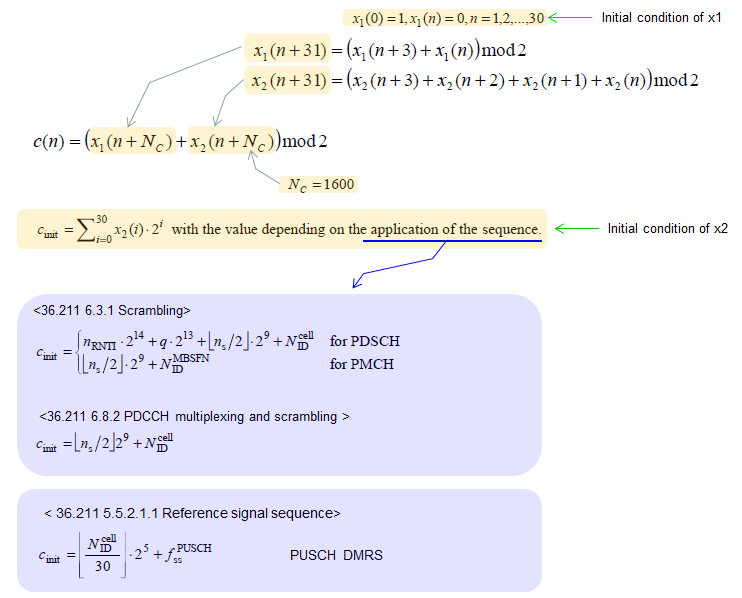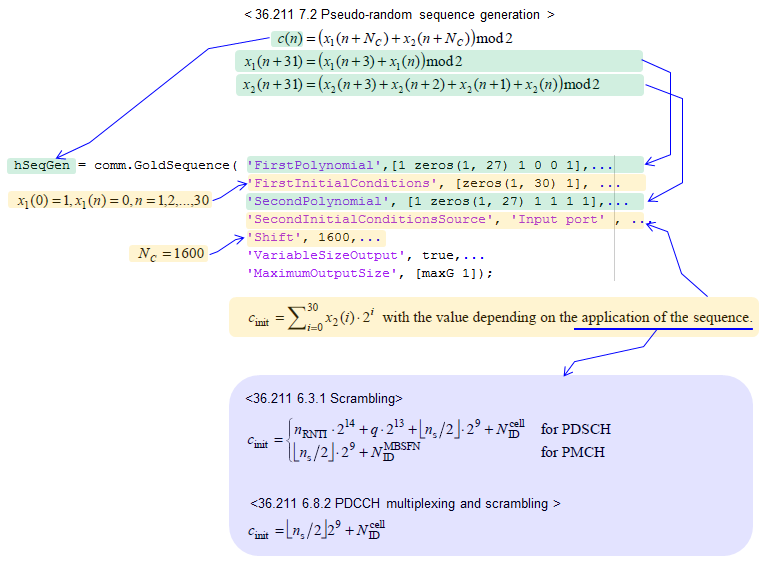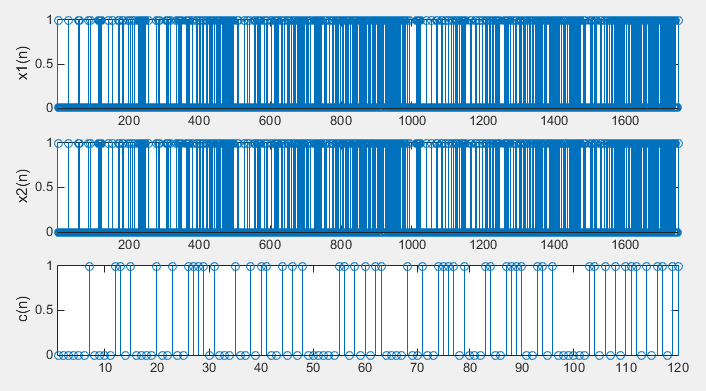LTE Quick Reference Pseudo-Random Sequence (Gold Sequence)   This is not a specific physical channel, but this sequence (variation of the sequence) are used in many way to generate a specific sequence itself (e.g, Downlink Reference Signal) or to scramble the data of a specific channel. The Pseudo-Random Sequence used for LTE is a type of Gold Sequence defined as follows in 36.211 7.2 Pseudo-random sequence generation. (If you are not familiar with the concep of Gold Sequence, refer to Gold Code page)What is this used for ?   What are we use this for ? There are several different application for this sequence as listed below. Generation of Downlink Reference Signal : Two of this Pseudo Random Sequence (Gold Sequence) is used Scrambling of PDSCH Scrambling of PMCH Scrambling of PDCCH Generation of PUSCH DMRS (e.g, PUSCH DMRS - 1RB)     How to implement ?   I will show you a couple of different ways of implementing this pseudo random sequence here. Depending on the language that you use and your programming style, you would have a little bit different code. Matlab communication package and srsLTE API is verified code, but my MatLab code is not verified but I hope it is written correctly.   < Matlab Communication Package >   If you have access to Matlab Communication Toolbox, you can implement this sequence as shown below. (This Matlab code clip is from the book : Understanding LTE with Matlab)< srsLTE >   Following is the implementation in srsLTE.   void srslte_sequence_set_LTE_pr(srslte_sequence_t *q, uint32_t seed) {   int n;   uint32_t *x1, *x2;     x1 = calloc(Nc + q->len + 31, sizeof(uint32_t));   if (!x1) {     perror("calloc");     return;   }   x2 = calloc(Nc + q->len + 31, sizeof(uint32_t));   if (!x2) {     free(x1);     perror("calloc");     return;   }     for (n = 0; n < 31; n++) {     x2[n] = (seed >> n) & 0x1;   }   x1 = 1;     for (n = 0; n < Nc + q->len; n++) {     x1[n + 31] = (x1[n + 3] + x1[n]) & 0x1;     x2[n + 31] = (x2[n + 3] + x2[n + 2] + +x2[n+1] + x2[n]) & 0x1;   }     for (n = 0; n < q->len; n++) {     q->c[n] = (x1[n + Nc] + x2[n + Nc]) & 0x1;   }     free(x1);   free(x2); }     < My own MatLab code >   Following is the Matlab code that I wrote using Matlab default function only. This would be very inefficient at machine level. You may not see this kind of implement at professional code example. But I wrote the code to make it look as closer to the way described in 3GPP document as possible. The purpose of this code is to give you better understanding of the 3GPP spec.   clear all;   % Initial condition of the polynomia x1(). This is fixed value as described in 36.211 7.2 x1_init = [1 0 0 0 0 0 0 0 0 0 ...            0 0 0 0 0 0 0 0 0 0 ...            0 0 0 0 0 0 0 0 0 0 ...            0];   % Initial condition of the polynomia x2(). % This is supposed to be used as c_init as described in 36.211 7.2 % But for simplicity, I just se the arbitrary value for now x2_init = [0 0 0 0 0 0 1 0 0 0 ...            0 0 0 0 0 0 0 0 0 0 ...            0 0 0 0 0 0 0 0 0 0 ...            0];   % Mpn is the length of the final sequence c() Mpn = 12*10;   % Nc as defined in 36.211 7.2 Nc = 1600;   % Create a vector(array) for x1() and x2() all initialized with 0 x1 = zeros(1,Nc + Mpn + 31); x2 = zeros(1,Nc + Mpn + 31);   % Create a vector(array) for c() all initialized with 0 c = zeros(1,Mpn);   % Initialize x1() and x2() x1(1:31) = x1_init; x2(1:31) = x2_init;   % generate the m-sequence : x1() for n = 1 : (Mpn+Nc)    x1(n+31) = mod(x1(n+3) + x1(n),2); end;   % generate the m-sequence : x2() for n = 1 : (Mpn+Nc)    x2(n+31) = mod(x2(n+3) + x2(n+2) + x2(n+1) + x2(n),2); end;   % generate the resulting sequence (Gold Sequence) : c() for n = 1 : Mpn     c(n)= mod(x1(n+Nc) + x2(n+Nc),2); end;     % Following has no meaning in practice, but I just plotted the result just to give you % overall pattern   subplot(3,1,1); stem(x1);xlim([1 length(x1)]); ylabel('x1(n)');   subplot(3,1,2); stem(x2);xlim([1 length(x2)]); ylabel('x2(n)');     subplot(3,1,3); stem(c);xlim([1 length(c)]); ylabel('c(n)');